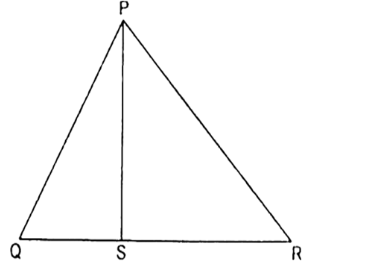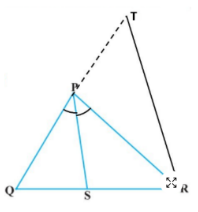Courses
Courses for Kids
Free study material
Free LIVE classes
MoreLIVE
Join Vedantu’s FREE Mastercalss

# In figure, PS is the bisector of $\angle QPR$ of $\vartriangle PQR.$ Prove that $\dfrac{{QS}}{{SR}} = \dfrac{{PQ}}{{PR}}$Verified
361.8k+ views
Hint: We are going to use basic proportionality theorem, Alternate interior angles and corresponding angles to solve this problem.

In $\vartriangle QRT,RT\parallel SP$ [By construction]
And PS intersects QT and QR at two distinct points P and Q.
Therefore, QT and QR will be divided in the same ratio.

$\dfrac{{QS}}{{SR}} = \dfrac{{PQ}}{{PT}}$ ..... (1) [Basic proportionality theorem]
Now $RS\parallel SP$ and PR is transversal.

Therefore, $\angle SPR = \angle PRT$.....(2) [Alternate interior angles]
And $\angle QPS = \angle PTR$..... (3) [Corresponding angles]
Also given that PS is the bisector of $\angle QPR.$
$\Rightarrow \angle QPR = \angle SPR$
From equations (2) and (3) $\angle PTR = \angle PRT$
Therefore, PT = PR [Sides opposite to equal angles of a triangle are equal]
Putting PT= PR in equation (1)
$\dfrac{{QS}}{{SR}} = \dfrac{{PQ}}{{PR}}$

Hence proved.Note: Basic proportionality theorem states that if a line is drawn parallel to one side of a triangle, intersecting the other two sides at distinct points, then the other two sides are divided in the same ratio.
Last updated date: 20th Sep 2023
Total views: 361.8k
Views today: 5.61k